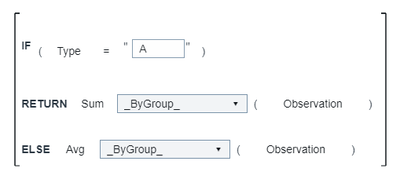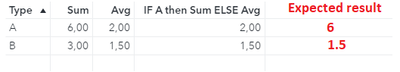## Conditional aggregation of a single measurement in Visual Analytics(3.5)

Hi!

I'm working on a Visual Analytics report that utilize the Suppress function when calculating different measurements (If there is less than 5 observations then suppress). The end user of the report now wants to add an additional level on this logic since suppression is only required for a specific category of observations, simply put,

IF Type=B and number of observations < 5 then Suppress ELSE "calculate measurement". I've been prying at this for weeks but haven't manage to get it to work thus I wonder, is this logic possible?

Below is a simplified example

Let's establish some sample data:

 Type Observation A 1 A 2 A 3 B 1 B 2

Imagine that I want to create a conditional Sum calculation of Observation that is always allowed to be shown when Type="A" but can't be shown when Type="B" In this case the desired result would be:

 Type Conditional Sum A 6 B *

Suppression is an aggregated measurement so lets simplify the problem:
Is it possible in Visual Analytics to create a measurement that is the Sum of Observation when Type="A" and average of Observation when Type="B"?

I've tried to express this in Visual Analytics as such:However, when I use this calculated measurement in a table I get the following result:In fact, whenever I try to use IF/ELSE logic in combination with a aggregated calculations it always results in the ELSE condition.

Conclusion
I understand that the IF logic expressed above evaluates each row in the data while the Sum and Avg is performed "by each group" and thus it's kind of doing two very different things.
I'm guessing what I'm looking for is something in the line of "IF current _ByGroup_ (Type) = "A" RETURN ..... ELSE ....." however I haven't found any operators that does that.

While the problem sounds simple, at this point I'm prepared to call it impossible due to how _ByGroups_ works but I'm hoping that I might be wrong 😄

Best regards and thanks in advance for any suggestions!

//Winter